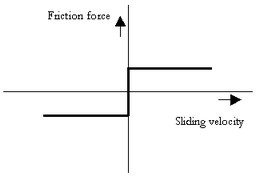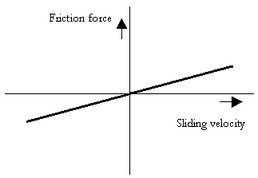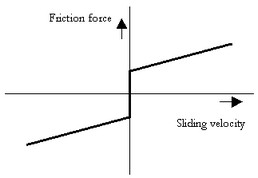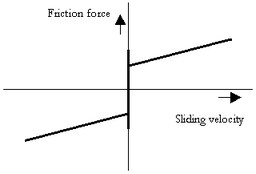﻿ 20-sim webhelp > Library > Iconic Diagrams > Mechanical > Rotation > Components > friction

# friction

Iconic Diagrams\Mechanical\Rotation\Components

C

V

CV

SCVS

LuGre

## Use

Domains: Continuous. Size: 1-D. Kind: Iconic Diagrams (Rotation).

## Introduction

The friction model represent bearing friction with various friction models. The model have only one initial port p defined. Because any number of connections can be made, successive ports are named p1, p2, p3 etc. 20-sim will automatically create equations such that the resulting force p.F is equal to the sum of the forces of all connected ports p1 .. pn. The velocities of all connected ports are equal to p.v.

p.F = sum(p1.F, p2.F, ....)

p.v = p1.v = p2.v = ....

## Description - C

This model represents a bearing with friction torque described as coulomb friction:

p.T = Tc*tanh(slope*p.omega);

Tc: the coulomb friction

slope: the steepness of the coulomb friction curve.## Interface - C

 Ports Description p[any] Any number of connections can be made (Rotation). Causality Fixed torque out Parameters Tc slope Coulomb friction [N.m] Steepness of Coulomb friction curve [s/rad]

## Description - V

This model represents a bearing with friction force described as viscous friction:

p.T = d*p.omega;

d: the viscous damping## Interface - V

 Ports Description p[any] Any number of connections can be made (Rotation). Causality Fixed torque out Input Fn Normal force [N] Parameters d Viscous friction torque or damping [N.m.s/rad]

## Description - CV

This model represents a bearing with friction force described as coulomb plus viscous friction:

p.T = Tc*tanh(slope*p.omega) + d*p.omega;

d: the viscous damping

Tc: the coulomb friction

slope: the steepness of the coulomb friction curve.## Interface - CV

 Ports Description p[any] Any number of connections can be made (Rotation). Causality Fixed torque out Input Fn Normal force [N] Parameters mu_v Tc slope Viscous damping [N.m.s/rad] Coulomb friction [N.m] Steepness of Coulomb friction curve [s/rad]

## Description - SCVS

This model represents a bearing with friction force described as static plus coulomb plus viscous plus Stribeck friction:

p.T = (( Tc + (Tst*abs(tanh( slope*p.omega )) - Tc)

* exp( -((p.omega / v_st)^2 )) ) * sign(p.omega)

+ d * p.omega);

Tst: the static friction

d: the viscous damping

Tc: the coulomb friction

slope: the steepness of the coulomb and static friction curve.

v_st: the characteristic Stribeck velocity.## Interface - SCVS

 Ports Description p[any] Any number of connections can be made (Rotation). Causality Fixed torque out Input Fn Normal force [N] Parameters Tst d Tc slope v_st Static friction torque [N.m] Viscous friction torque or damping [N.m.s/rad] Coulomb friction [N.m] Steepness of Coulomb friction curve [s/rad] Characteristic Stribeck velocity [rad/s]

## Description - LuGre

This model represents a bearing with friction force described by the LuGre friction model:

p.T = f_lg(p.omega);

f_lg: the LuGre friction model

## Interface- LuGre

Ports

Description

p[any]

Any number of connections can be made (Rotation).

Causality

Fixed torque out

Input

Fn

Normal force [N]

## Parameters

Tc

Tst

d

v_st

c

Coulomb friction [N.m]

Static friction torque [m]